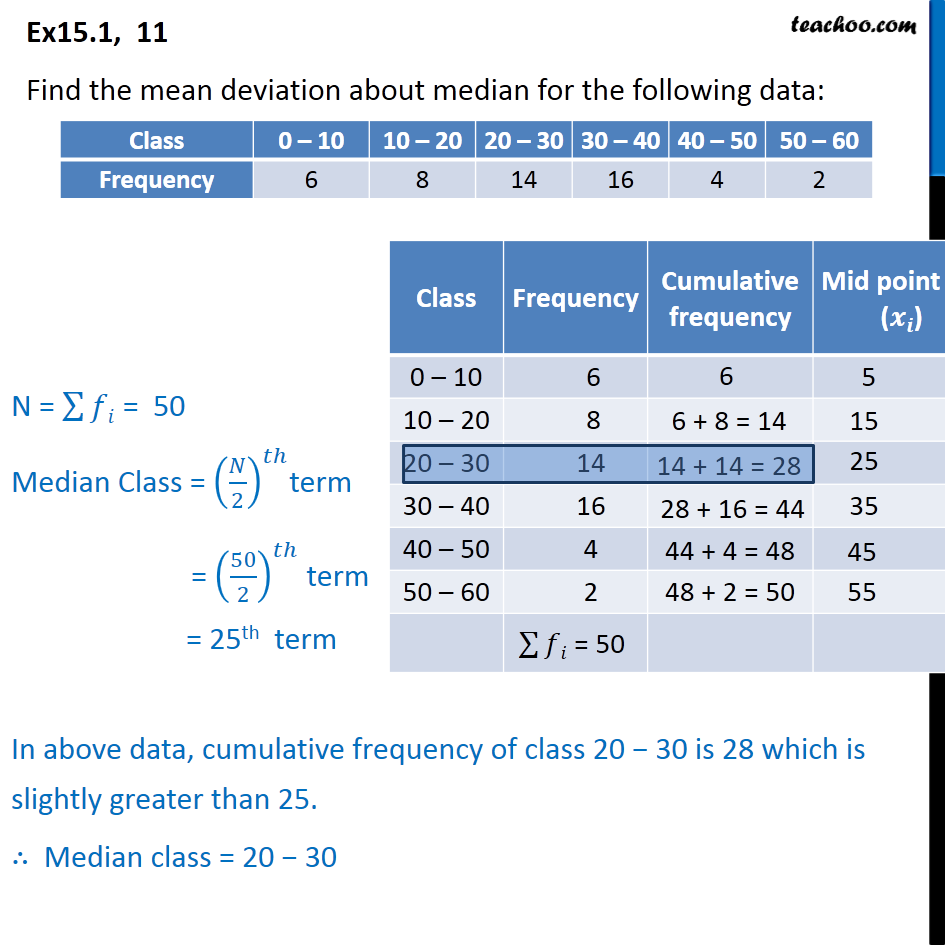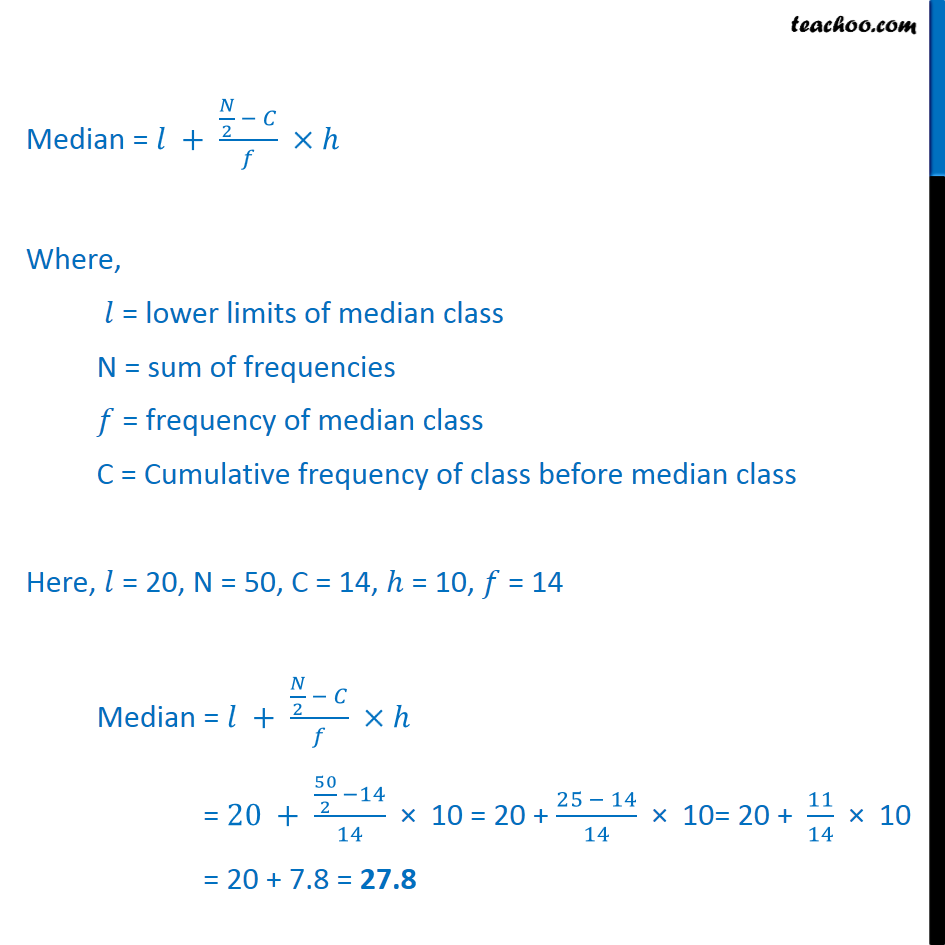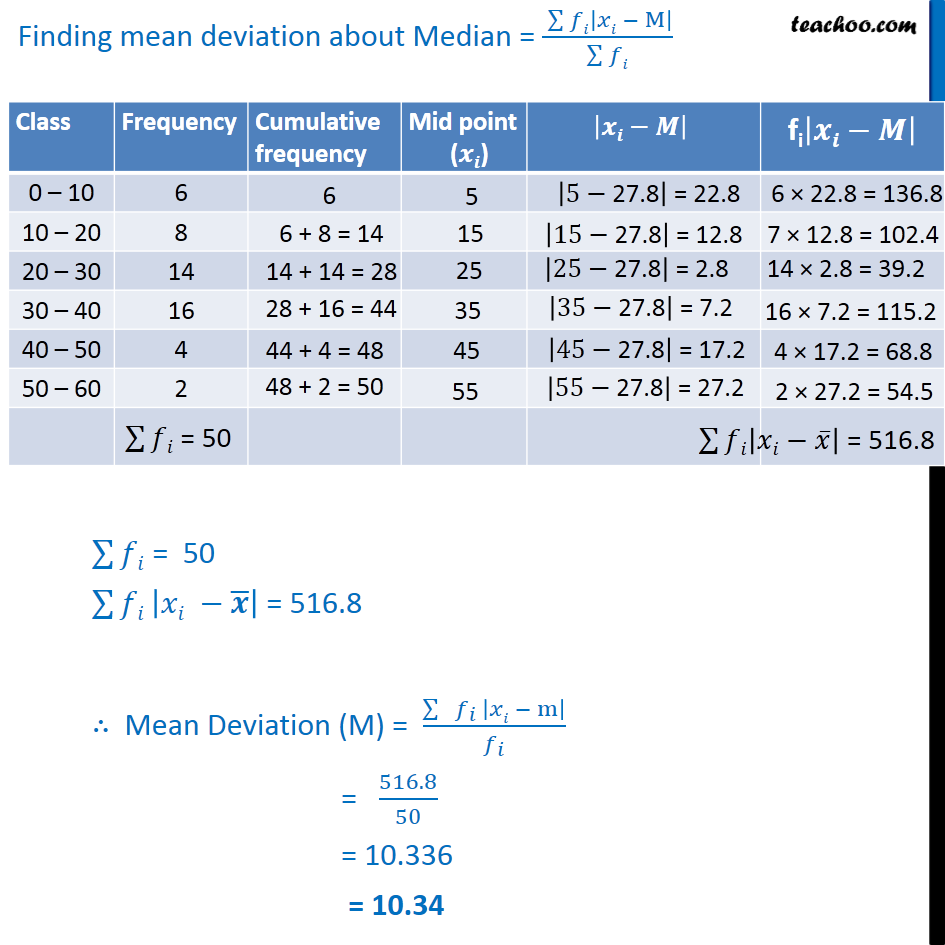1. Chapter 15 Class 11 Statistics (Term 1)
2. Serial order wise
3. Ex 15.1

Transcript

Ex 15.1, 11 Find the mean deviation about median for the following data: Median = + ( /2 )/ Where, = lower limits of median class N = sum of frequencies = frequency of median class C = Cumulative frequency of class before median class Here, = 20, N = 50, C = 14, = 10, = 14 Median = + ( /2 )/ = 20 + ( 50/2 14)/14 10 = 20 + (25 14)/14 10= 20 + 11/14 10 = 20 + 7.8 = 27.8

Ex 15.1

Chapter 15 Class 11 Statistics (Term 1)
Serial order wise